Next: Problem Up: Physical Background Previous: Problem   Contents

# Free-fall Time

If the pressure force can be neglected in the equation of motion (A.1), the gravitational force remains. Assuming the spherical symmetry, consider the gravity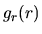at the position of radial distance from the center being equal to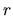. Using the Gauss' theorem,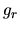is related to the mass inside of, which is expressed by the equation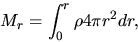(2.12)

andis written as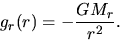(2.13)

This leads to the equation motion for a cold gas under a control of the self-gravity is written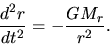(2.14)

Analyzing the equation is straightforward, multiplying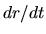gives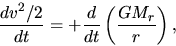(2.15)

which leads to the conservation of mechanical energy as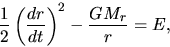(2.16)

in which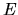represents the total energy of the pressureless gas element and it is fixed from the initial condition. If the gas is static initially at the distance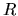, the total energy is negative as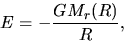(2.17)

because at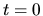,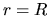and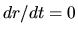.

The solutions of equation (2.16) are well known as follows:

1. the case of negative energy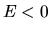. Considering the case that the gas sphere is inflowing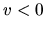, equation (2.16) becomes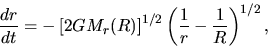(2.18)

where we assumed initiallyat. Using a parameter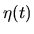, the radius of the gas element at some epoch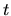is written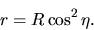(2.19)

In this case, equation (2.18) reduces to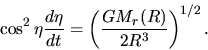(2.20)

This gives us the expression ofas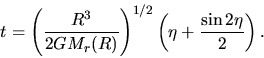(2.21)

This corresponds to the closed universe in the cosmic expansion (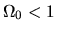).
2. if the energy is equal to zero, the solution of equation (2.16) is written as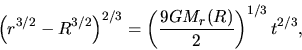(2.22)

where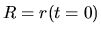.

SubsectionsNext: Problem Up: Physical Background Previous: Problem   Contents
Kohji Tomisaka 2007-07-08